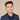# Pick Random Element From Array In Swift

To get a random element from an array you can use the `randomElement()` function for arrays.

Here is a code example:

``````let numbers = [5, 2, 9, 11, 20, 3030]

if let random = numbers.randomElement() {
print("The random number is \(random).")
}``````

The reason we need the `if let` is because `randomElement()` returns an optional. It will return nil if your array is empty.

Here’s an example where it will return `nil`:

``````let emptyArray: [Int] = []
print(emptyArray.randomElement()) // nil``````

## String Example

This also works with any type of array, here’s an example of a `String` array:

``````let names = "Eddy, Edson, Chika, Manju, Hamilton, Amber, Disha, Soumya, Rohit"
if let random = names.randomElement() {
print("The random name is \(random).")
}``````
If you liked this post and want to learn more, check out The Complete iOS Developer Bootcamp. Speed up your learning curve - hundreds of students have already joined. Thanks for reading!## Eddy Chung

I teach iOS development on ZeroToAppStore.com.